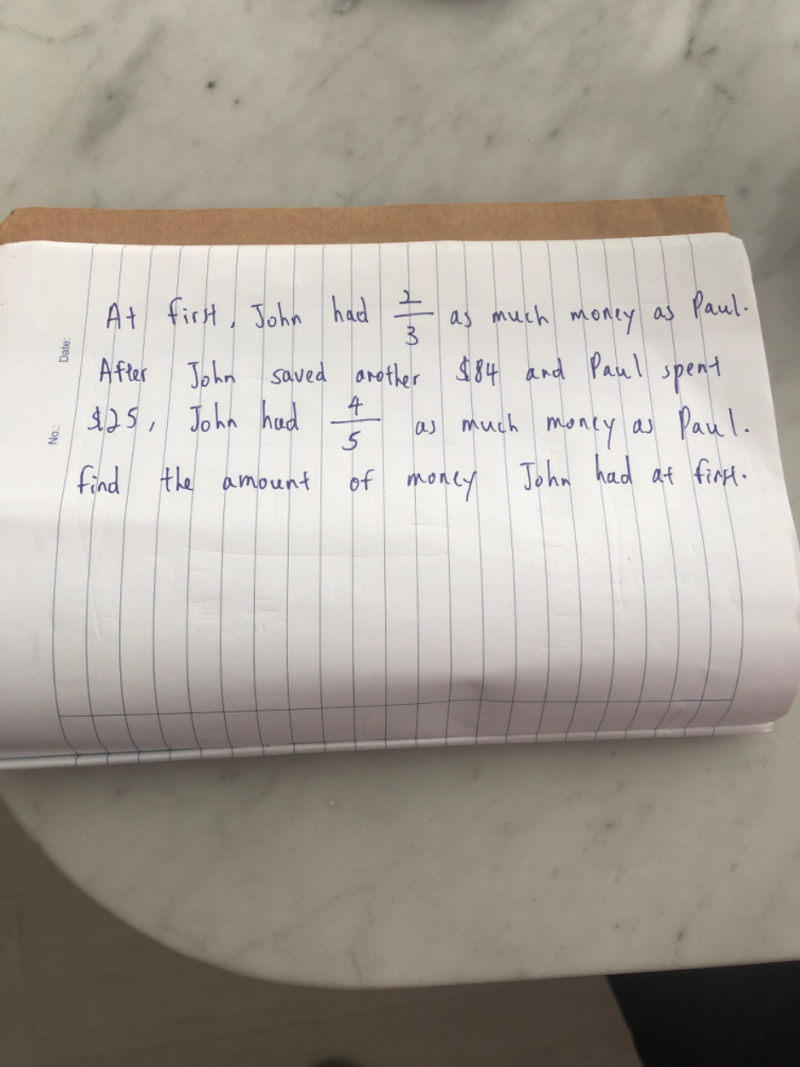# QuestionPls help. Thanks

John                          Paul

At first            2u  x5 = 10u              3u x 4   = 12u

After              +84 x 5 = 420           -25 x 4 = -100

New                 4p x5 = 20p              5p x 4 = 20p

10u + 420 = 12u -100

2u = 520

Hence John has \$520 at first.